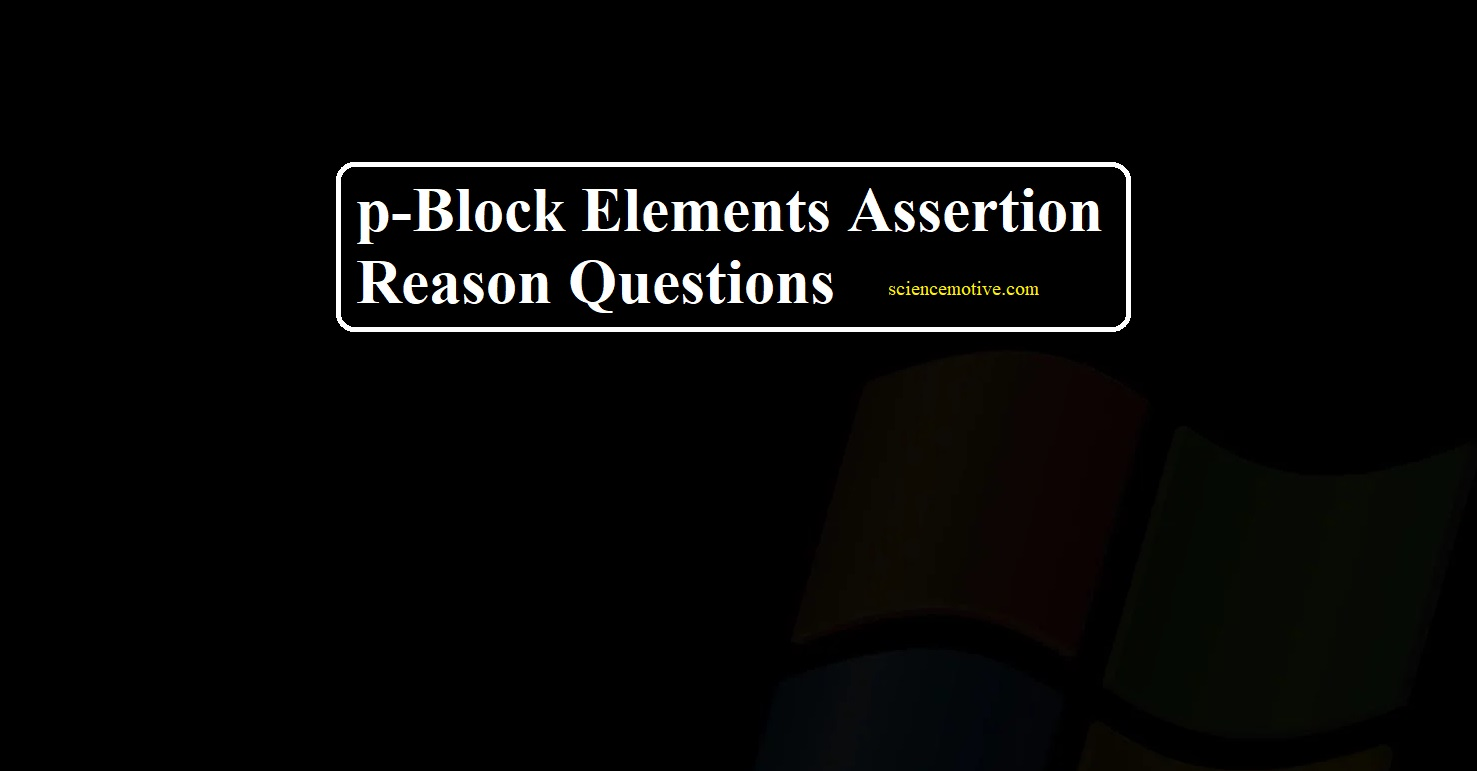# p-Block Elements Assertion Reason Questions# p-Block Elements Assertion Reason Questions

p-Block Elements Assertion Reason Questions

Q 1. Assertion: Hydrolysis of XeF6 is an example of a redox reaction.
Reason: XeF6 when hydrolysed yields XeOF4 and XeO2F2
Ans 1. (d) Assertion is wrong but the reason is the correct statement

Q 2. Assertion: The O-O bond length in ozone is identical to that in molecular oxygen.
Reason: The ozone molecule is a resonance hybrid of two canonical structures.
Ans 2. (a) Both A and R are true but R is the correct explanation of A

Q 3. Assertion: HI cannot be prepared by the action of conc. H2SO4 on KI.
Reason: HI is more volatile than H2SO4
Ans 3. (b) Both A and R are true but R is not a correct explanation of A

Q 4. Assertion: Boric acid behaves as a weak monobasic acid.
Reason: Boric acid contains hydrogen bonds in its structure.
Ans 4. (b) Both A and R are true but R is not a correct explanation of A

Q 5. Assertion: HCIO is a stronger acid than HBrO.
Reason: Greater is the electronegativity of the halogen, greater will attraction of electron pair towards it and hence more easily the H+ ion will be released.
Ans 5. (a) Both A and R are true but R is the correct explanation of A

Q 6. Assertion: SO3 has a planer structure
Reason: S atom in SO3 is sp2 hybridized and O-S-O bond angle is 1200.
Ans 6. (a) Both A and R are true but R is the correct explanation of A

Q 7. Assertion: Salts of ClO3 and ClO4are well known but those of FO3 and FO4 are non-existent.
Reason: F is more electronegative than O while Cl is less electronegative than O.
Ans 7. (b) Both A and R are true but R is not a correct explanation of A

Q 8. Assertion: Both rhombic and monoclinic sulphur exist as S8 but oxygen exists as O2.
Reason: Oxygen forms pπ – pπ multiple bonds due to small size and small bond lengths but pπ – pπ bonding is not possible in sulphur.
Ans 8. (a) Both A and R are true but R is the correct explanation of A

Q 9. Assertion: NaCl reacts with concentrated H2SO4 to give colourless fumes with a pungent smell. But on adding MnO2 the fumes become greenish-yellow.
Reason: MnO2 oxidises HCl to chlorine gas which is greenish-yellow.
Ans 9. (a) Both A and R are true but R is the correct explanation of A

Q 10. Assertion: SF6 cannot be hydrolysed but SF4 can be
Reason: Six F atoms in SF6 prevent the attack of H2O on the sulphur atom of SF6.
Ans 10. (a) Both A and R are true but R is the correct explanation of A.

p-Block Elements Assertion Reason Questions